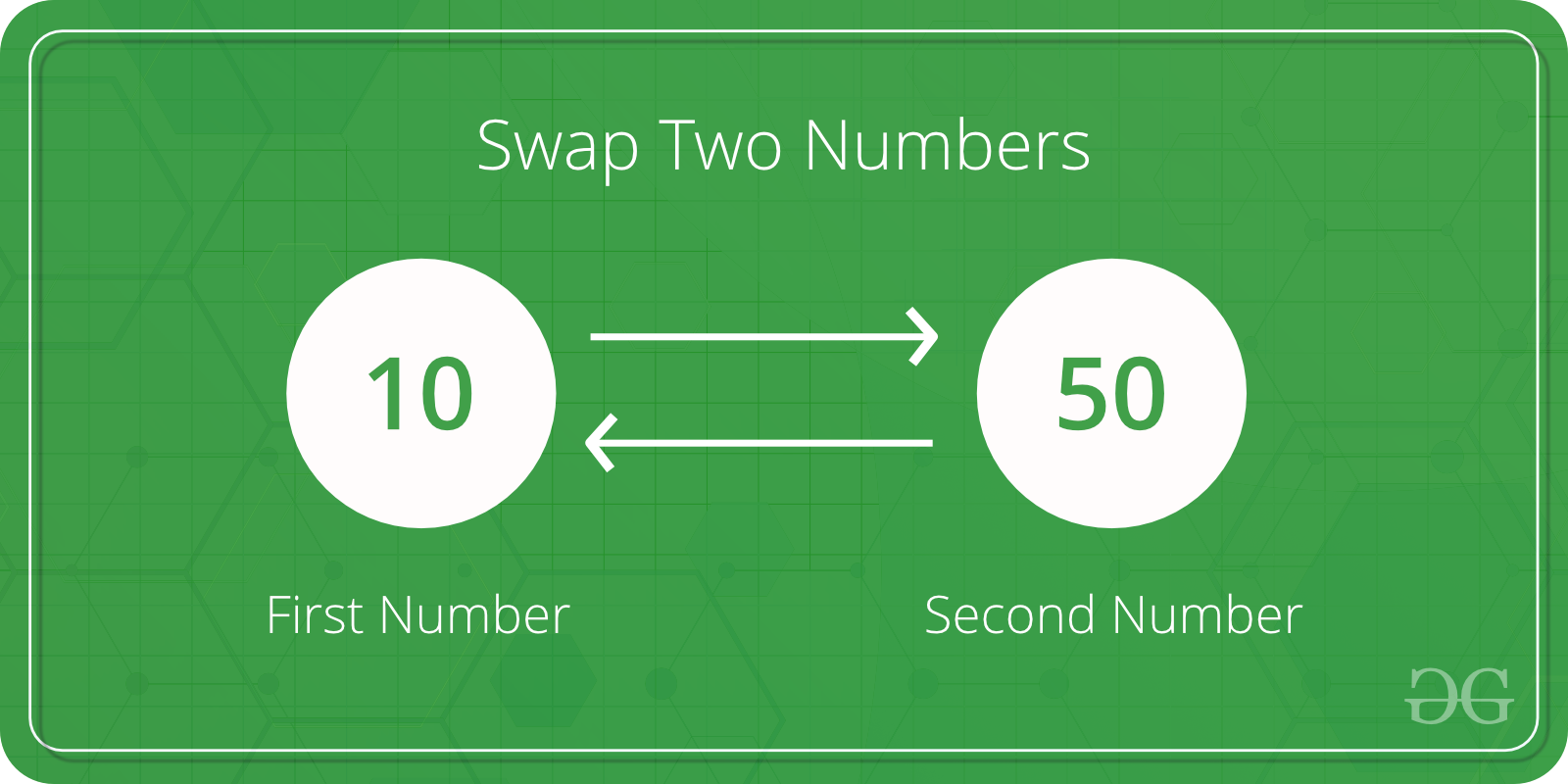Open in App
Not now

# C Program to Swap two Numbers

• Difficulty Level : Basic
• Last Updated : 05 Dec, 2022

Given two numbers, write a C program to swap the given numbers.

```Input : x = 10, y = 20;
Output : x = 20, y = 10

Input : x = 200, y = 100
Output : x = 100, y = 200```The idea is simple

1. Assign x to a temp variable : temp = x
2. Assign y to x : x = y
3. Assign temp to y : y = temp

Let us understand with an example.

x = 100, y = 200 After line 1: temp = x temp = 100 After line 2: x = y x = 200 After line 3 : y = temp y = 100

## C

 `// C program to swap two variables` `#include `   `int` `main()` `{` `    ``int` `x, y;` `    ``printf``(``"Enter Value of x "``);` `    ``scanf``(``"%d"``, &x);` `    ``printf``(``"\nEnter Value of y "``);` `    ``scanf``(``"%d"``, &y);`   `    ``int` `temp = x;` `    ``x = y;` `    ``y = temp;`   `    ``printf``(``"\nAfter Swapping: x = %d, y = %d"``, x, y);` `    ``return` `0;` `}`

Output:

```Enter Value of x 12

Enter Value of y 14

After Swapping: x = 14, y = 12 ```

Time Complexity: O(1)
Auxiliary Space: O(1)

How to write a function to swap? Since we want the local variables of main to modified by swap function, we must them using pointers in C

## C

 `// C program to swap two variables using a ` `// user defined swap()` `#include `   `// This function swaps values pointed by xp and yp` `void` `swap(``int` `*xp, ``int` `*yp)` `{` `    ``int` `temp = *xp;` `    ``*xp = *yp;` `    ``*yp = temp;` `}`   `int` `main()` `{` `    ``int` `x, y;` `    ``printf``(``"Enter Value of x "``);` `    ``scanf``(``"%d"``, &x);` `    ``printf``(``"\nEnter Value of y "``);` `    ``scanf``(``"%d"``, &y);` `    ``swap(&x, &y);` `    ``printf``(``"\nAfter Swapping: x = %d, y = %d"``, x, y);` `    ``return` `0;` `}`

Output:

```Enter Value of x 12

Enter Value of y 14

After Swapping: x = 14, y = 12 ```

Time Complexity: O(1)
Auxiliary Space: O(1)

How to do in C++? In C++, we can use references also.

## CPP

 `// C++ program to swap two variables using a ` `// user defined swap()` `#include `   `// This function swaps values referred by ` `// x and y,` `void` `swap(``int` `&x, ``int` `&y)` `{` `    ``int` `temp = x;` `    ``x = y;` `    ``y = temp;` `}`   `int` `main()` `{` `    ``int` `x, y;` `    ``printf``(``"Enter Value of x "``);` `    ``scanf``(``"%d"``, &x);` `    ``printf``(``"\nEnter Value of y "``);` `    ``scanf``(``"%d"``, &y);` `    ``swap(x, y);` `    ``printf``(``"\nAfter Swapping: x = %d, y = %d"``, x, y);` `    ``return` `0;` `}`

Output:

```Enter Value of x 12

Enter Value of y 14

After Swapping: x = 14, y = 12 ```

Time Complexity: O(1)
Auxiliary Space: O(1)

Is there a library function? We can use C++ library swap function also.

## CPP

 `// C++ program to swap two variables using a ` `// user defined swap()` `#include ` `using` `namespace` `std;`   `int` `main()` `{` `    ``int` `x, y;` `    ``printf``(``"Enter Value of x "``);` `    ``scanf``(``"%d"``, &x);` `    ``printf``(``"\nEnter Value of y "``);` `    ``scanf``(``"%d"``, &y);` `    ``swap(x, y);` `    ``printf``(``"\nAfter Swapping: x = %d, y = %d"``, x, y);` `    ``return` `0;` `}`

Output:

```Enter Value of x 12

Enter Value of y 14

After Swapping: x = 14, y = 12 ```

Time Complexity: O(1)
Auxiliary Space: O(1)

Swapping two numbers without using a temporary variable:

Approach: the simple idea behind this code is to use arithmetic operators. We will take the sum of the two numbers and store it in one number and store the difference of both the numbers in the other number. Finally, we will store the difference of both the numbers in the first number. Since the values will be updated after the first two operations we will be able to swap the numbers.

Algorithm:

1) Take the input of the two numbers.
2) Store the sum of both the numbers in the first number and store the difference of both the numbers in the second number.
3) Finally store the difference of both the numbers in the first number and print both the numbers.

Below is the implementation:

## C

 `// C program to swap two numbers without using a third` `// variable` `#include ` `int` `main()` `{` `    ``int` `a, b;` `    ``printf``(``"Enter the two numbers : \n"``);` `    ``scanf``(``"%d %d"``, &a, &b);` `    ``printf``(``"Before swapping A is : %d and B is %d \n"``, a,` `           ``b);` `    ``a = a + b;` `    ``b = a - b;` `    ``a = a - b;` `    ``printf``(``"After swapping A is : %d and B is : %d \n"``, a,` `           ``b);` `    ``return` `0;` `}`

## C++

 `// C++ program to swap two numbers without using a third` `// variable` `#include ` `using` `namespace` `std;` `int` `main()` `{` `    ``int` `a, b;` `    ``cout << ``"Enter the two numbers : \n"``;` `    ``cin >> a >> b;` `    ``cout << ``"Before swapping A is : "` `<< a` `         ``<< ``" and B is :  "` `<< b << ``"\n"``;` `    ``a = a + b;` `    ``b = a - b;` `    ``a = a - b;` `    ``cout << ``"After swapping A is : "` `<< a` `         ``<< ``" and B is :  "` `<< b << ``"\n"``;` `    ``return` `0;` `}`

Output

```Enter the two numbers :
Before swapping A is : 0 and B is 32767
After swapping A is : 32767 and B is : 0 ```

Time Complexity: O(1)
Auxiliary Space: O(1)

How to swap without using a temporary variable?

My Personal Notes arrow_drop_up
Related Articles